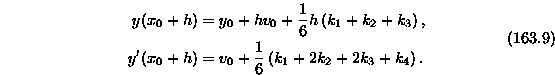Errors in the First Edition of
Handbook of Differential Equations

LAST UPDATED: April 21, 1998

NOTE THAT THERE MAY EXIST ADDITIONAL ERRORS IN THIS EDITION. PLEASE LOOK AT THE LIST OF ERRATA FOR LATER EDITIONS.

1.
On page 288, equation (75.1) is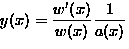. This is incorrect, it should have been (note the minus sign)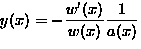2.
On page 289, equation (75.5) is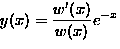. This is incorrect, it should have been (note the minus sign)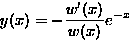3.
On page 376 the worked example uses the equation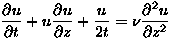. This equation is not solvable by similarity methods. The second edition used, as an example, the equation,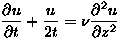.

4.
On page 577, equations (163.8) and (163.9) are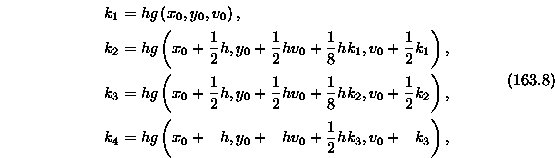and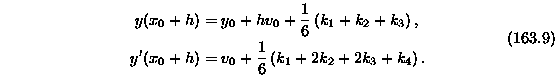They are incorrect, they should have been (note the second term in the defining equation for k3 , and the lack of an h in the equation for y(x0+h) ):and